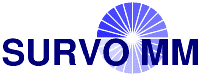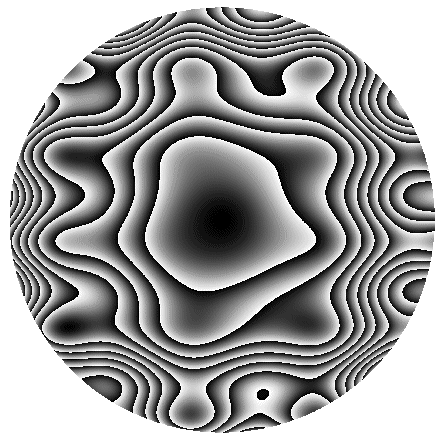# Environment for creative processing of text and numerical data## Front cover of the Survo User Guide

S.Mustonen, Survo Users' Guide (1992), p. 288### Edit field:

```   1 *
2 *`PLOT Z(X,Y)=if(R<1)then(A)else(2047/2048)`
3 *R=X*X+Y*Y A=R*(1+0.2*sin(10*X)+0.2*sin(15*Y))
4 *X=-1,1,0.002  ZSCALING=8,0
5 *Y=-1,1,0.002
6 *TYPE=CONTOUR DEVICE=PS
7 *SIZE=1500,1500 XDIV=0,1,0 YDIV=0,1,0 FRAME=0 HEADER=
8 *

```